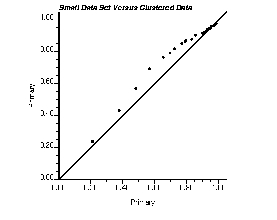GSLIB Help Page: QPPLTDescription:
• qpplt takes univariate data from two data files and generates either a Q-Q or a P-P plot. Q-Q plots compare the quantiles of two data distributions, and P-P plots compare the cumulative probabilities of two data distributions. These graphical displays are used to compare two different data distributions, e.g., compare an original data distribution to a distribution of simulated points.Parameters:
• datafl1: The data file for the first variable (x axis) in a simplified Geo-EAS format.
• ivr1 and iwt1: column number for the variable and the weight. If iwt1 less than or equal to 0, equal weighting is considered.
• datafl2: The data file for the second variable (y axis) in a simplified Geo-EAS format. Note: It is possible that datafl2 is the same as datafl1
• ivr2 and iwt2: column number for the variable and the weight. If iwt2 less than or equal to 0, equal weighting is considered.
• tmin and tmax: all values strictly less than tmin and strictly greater than tmax are ignored.
• outfl: file for PostScript output.
• qqorpp: The flag to tell the program whether to construct a Q-Q plot (qqorpp=0) or a P-P plot (qqorpp=1).
• npoints: number of points to use on the Q-Q or P-P plot (should not exceed the smallest number of data in datafl1 / datafl2
• xmin and xmax: minimum and maximum limits for x axis.
• ymin and ymax: minimum and maximum limits for y axis.
• ilog: =0 then an arithmetic scaling of the axes is used, =1 then a logarithmic scaling (base 10) is used. Note that the log scaling only applies to the Q-Q plot; the axis for the P-P plot are between 0 and 1
• title: a 40-character title for the top of the plot. A short description of the program